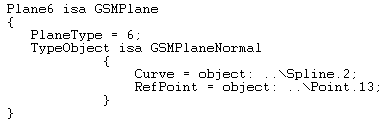# GSMPlane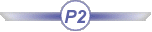## Definition:

A GSMPlane is a plane:

• generated by the Generative Shape Design product.
• available in the BasicWireFrame Package.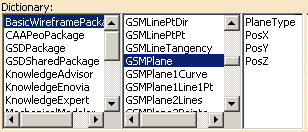## Attributes:

###### PlaneType

A plane is defined by its type. The attribute to use is PlaneType. The syntax to be used is: PlaneType = i, i corresponding to the type of plane that you want to create.
Please find below a table listing the existing types of planes that you can create and the digit to indicate.
 Plane Type in GSD Plane Type in the Package Corresponding digit Equation GSMPlaneEquation 0 Through 3 points GSMPlane3Points 1 Through 2 lines GSMPlane2Lines 2 Through a point and a line GSMPlane1line1Pt 3 Normal to a curve GSMPlane1Curve 4 Tangent to a surface GSMPlaneTangent 5 Normal to a plane GSMPlaneNormal 6

As mentionned above, you may create 7 different plane sub-types. Please find below a description of each sub-type, as well as its attributes and the syntax to use.

### Equation (GSMPlaneEquation)

The sub-type to be used in this case is GSMPlaneEquation which enables you to create a plane by using an equation. The following attributes are available for this sub-type:

 A (First component of the equation) B (Second component of the equation) C (Third component of the equation) Length RefPoint (point used to position the plane through this point)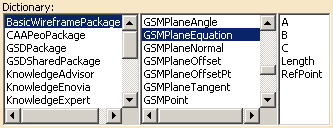These attributes can be combined as follows:

1st Combination 2nd Combination
• A which is defined by the syntax below:
`A=31; //A value is required`
• B which is defined by the syntax below:
`B=-47; //A value is required`
• C which is defined by the syntax below:
`C=-24; //A value is required`
• Length: enables the user to indicate the required length. It is defined by the syntax below:
`Length=24mm`
• A which is defined by the syntax below:
`A=31; //A value is required`
• B which is defined by the syntax below:
`B=-47; //A value is required`
• C which is defined by the syntax below:
`C=-24; //A value is required`
• RefPoint which is defined by the syntax below:
```RefPOint = object: ..\Point ;```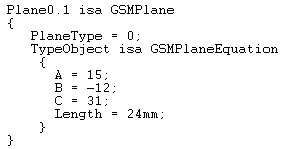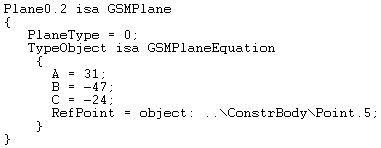### Through 3 points (GSMPlane3Points)

The sub-type to be used in this case is GSMPlane3Points which creates a plane passing through 3 points. The following attributes are available for this sub-type:

 Element1 (First point) Element2  (Second point) Element3 (Third point)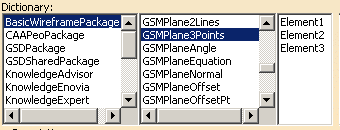These attributes can be combined as follows:

Combination
• Element1 which is defined by the syntax below:
`Element1 = object: ..\Point.1;`
• Element2 which is defined by the syntax below:
`Element2 = object: ..\Point.2;`
• Element3 which is defined by the syntax below:
`Element3 = object: ..\Point.3;`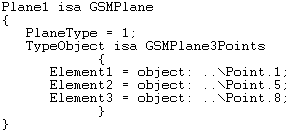### Through 2 Lines (GSMPlane2Lines)

The sub-type to be used in this case is GSMPlane2Lines which enables you to create a plane passing through 2 lines. The following attributes are available for this sub-type:

 Element1 (First line) Element2 (Second line)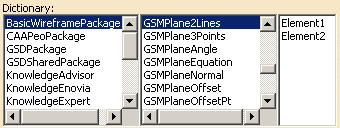Combination
• Element1 which is defined by the syntax below:
`Element1 = object: ..\Line.1;`
• Element2 which is defined by the syntax below:
`Element2 = object: ..\Line.2;`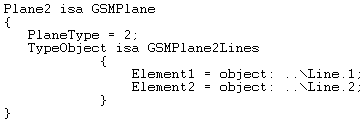### Through a Point and a Line (GSMPlane1line1Pt)

The sub-type to be used in this case is GSMPlane1Line1Pt which enables you to create a plane passing through a line and a point. The following attributes are available for this sub-type:

 Line: Line used to create the plane. RefPoint: Point used to create the plane.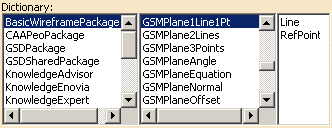The attributes must be used as follows:

Combination
• Line which is defined by the syntax below:
`Line = object: ..\Line.1;`
• RefPoint which is defined by the syntax below:
`RefPoint = object: ..\Point.2;`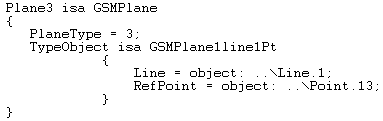### Normal to a Curve (GSMPlane1Curve)

The sub-type to be used in this case is GSMPlane1Curve which enables you to create a plane normal to a curve at a specified point.

 Element1: Line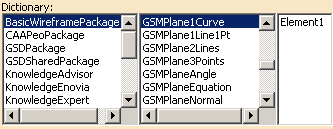This attribute is to be used as follows:

Combination
• Line which is defined by the syntax below:
`Line = object: ..\Spline.1;`

### Tangent to a Surface (GSMPlaneTangent)

The sub-type to be used in this case is GSMPlaneTangent which enables you to create a plane tangent to a surface at a specified point. The following attributes are available for this sub-type:

 RefPoint (Point) Support (Surface)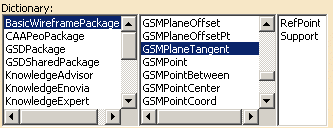These attributes are to be used as follows:

Combination
• Support which is defined by the syntax below:
`Support = object: ..\Spline.1;`
• RefPoint which is defined by the syntax below:
`RefPoint = object: ..\Point.4;`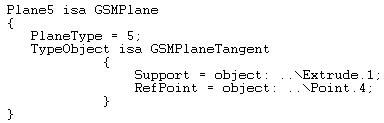### Normal to a Plane (GSMPlaneNormal)

The sub-type to be used in this case is GSMPlaneNormal. The following attributes are available for this sub-type:

 Curve: Reference curve used to create the plane. RefPoint: Reference point used to create the plane.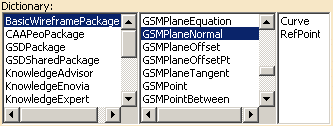These attributes are to be used as follows:

Combination
• Curve which is defined by the syntax below:
`Curve = object: ..\Spline.1;`
• RefPoint which is defined by the syntax below:
`RefPoint = object: ..\Point.4;`